# RS Aggarwal Solutions for Class 7 Maths Exercise 4C Chapter 4 Rational Numbers

RS Aggarwal Solutions for Class 7 Maths Exercise 4C Chapter 4 Rational Numbers in simple PDF are provided here. One topic covered in this exercise of RS Aggarwal Solutions for Class 7 Maths Chapter 4 Rational Numbers is the addition of rational numbers. Suppose we have to add two given rational numbers. First, convert each of them into rational numbers with a positive denominator. Students are advised to try solving the questions from RS Aggarwal Solutions for Class 7 Maths Exercise 4C Chapter 4 Rational Numbers.

## Download the PDF of RS Aggarwal Solutions For Class 7 Maths Chapter 4 Rational Numbers – Exercise 4C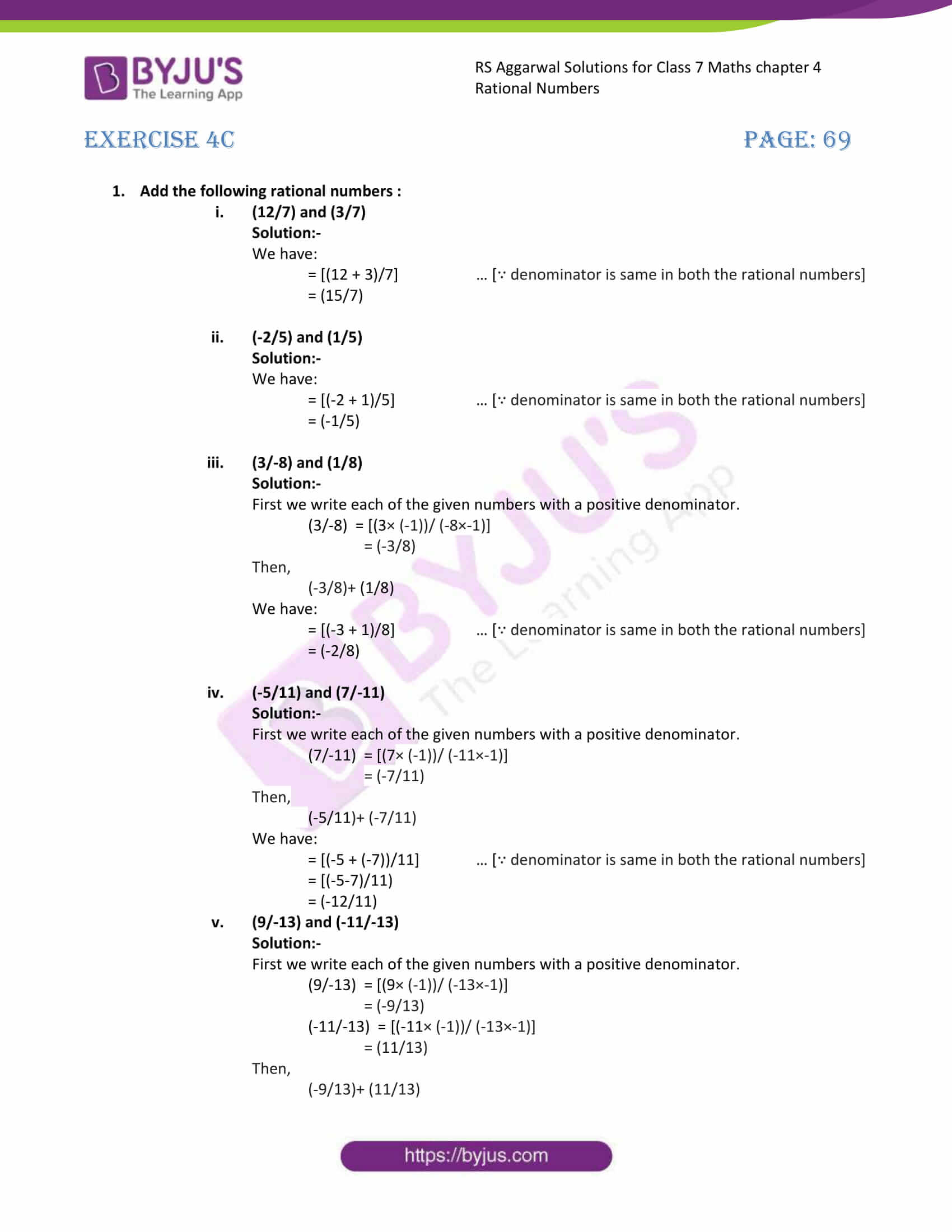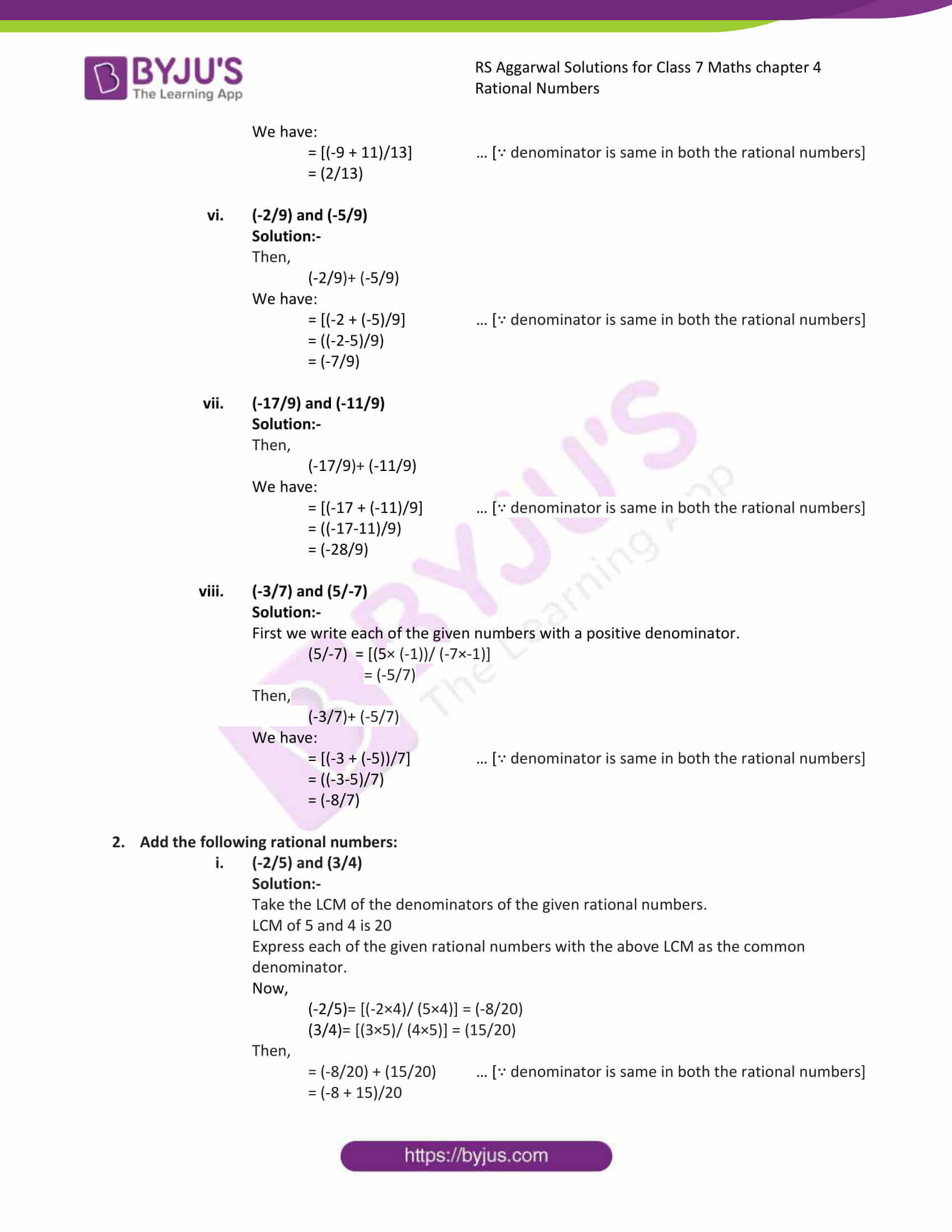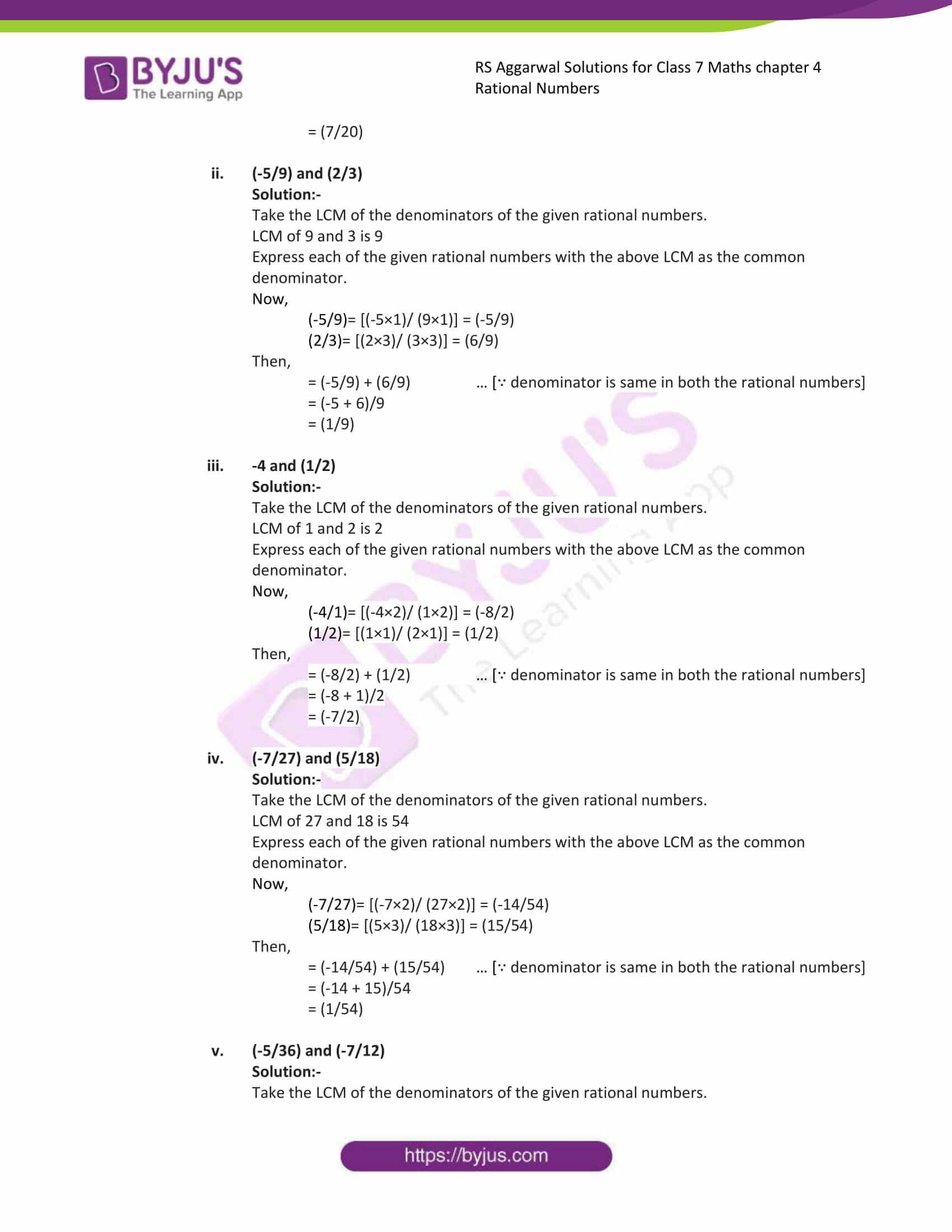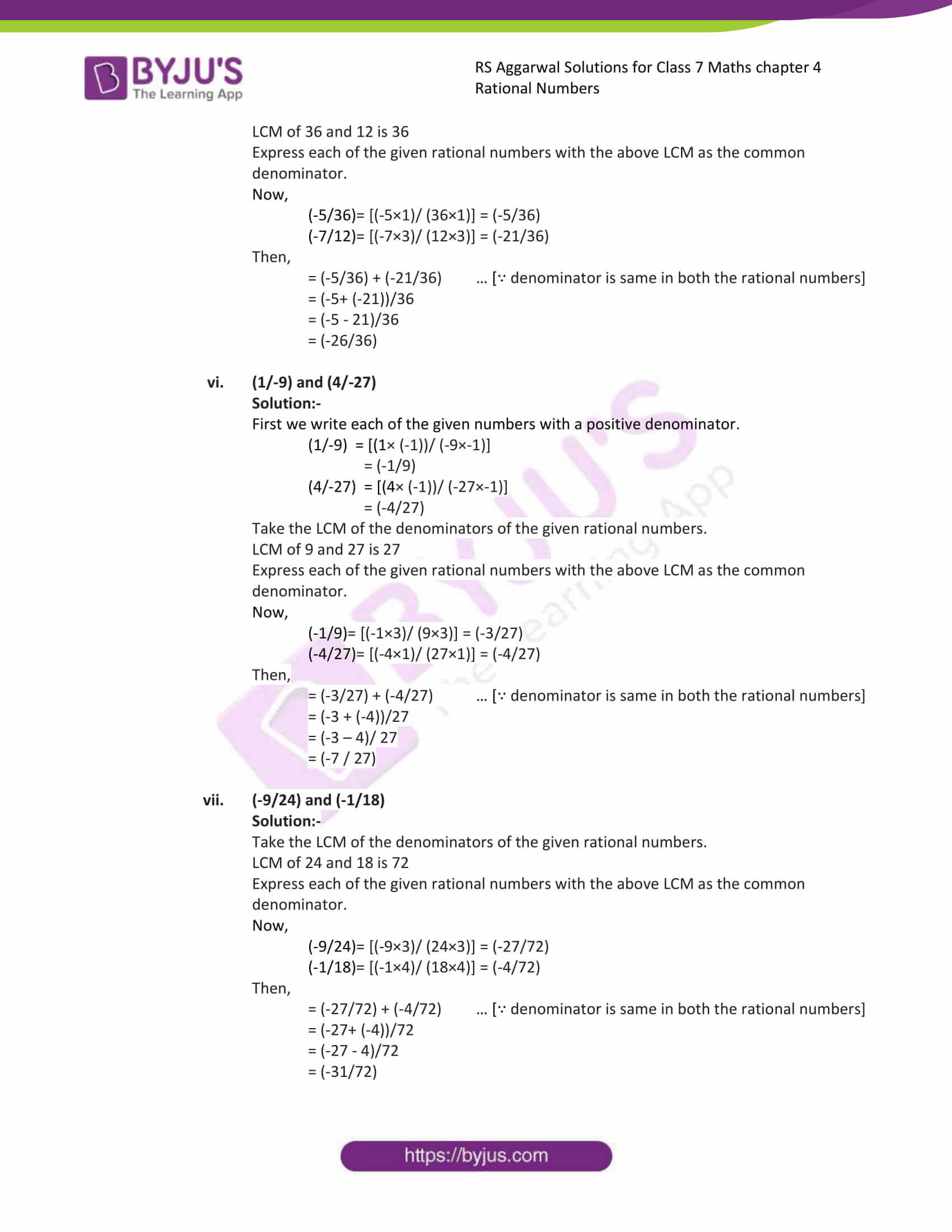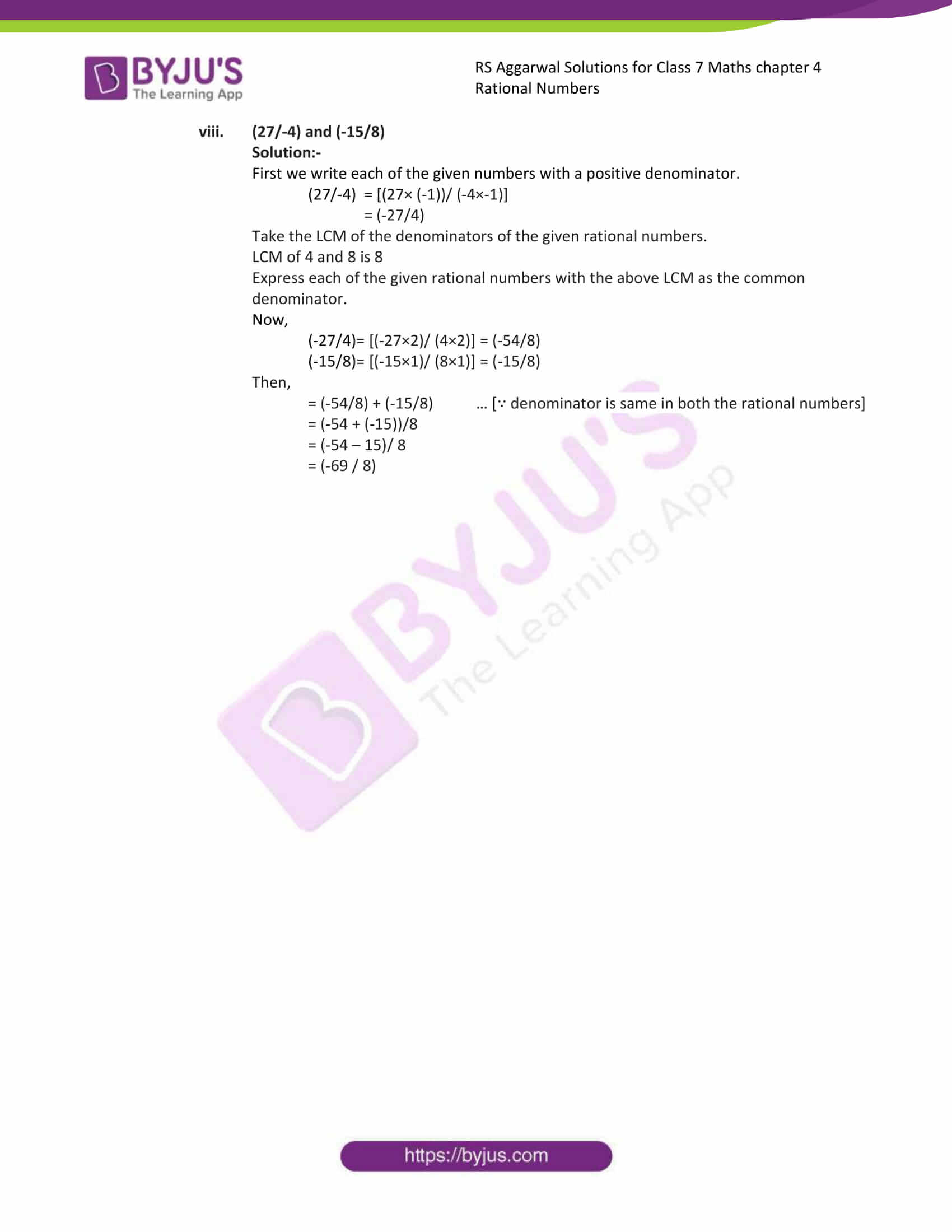### Access answers to Maths RS Aggarwal Solutions for Class 7 Chapter 4 – Rational Numbers Exercise 4C

1. Add the following rational numbers :
(i) (12/7) and (3/7)

Solution:-

We have:

= [(12 + 3)/7] … [∵ denominator is same in both the rational numbers]

= (15/7)

(ii) (-2/5) and (1/5)

Solution:-

We have:

= [(-2 + 1)/5] … [∵ denominator is same in both the rational numbers]

= (-1/5)

(iii) (3/-8) and (1/8)

Solution:-

First we write each of the given numbers with a positive denominator.

(3/-8) = [(3× (-1))/ (-8×-1)]

= (-3/8)

Then,

(-3/8)+ (1/8)

We have:

= [(-3 + 1)/8] … [∵ denominator is same in both the rational numbers]

= (-2/8)

(iv) (-5/11) and (7/-11)

Solution:-

First we write each of the given numbers with a positive denominator.

(7/-11) = [(7× (-1))/ (-11×-1)]

= (-7/11)

Then,

(-5/11)+ (-7/11)

We have:

= [(-5 + (-7))/11] … [∵ denominator is same in both the rational numbers]

= [(-5-7)/11)

= (-12/11)

(v) (9/-13) and (-11/-13)

Solution:-

First we write each of the given numbers with a positive denominator.

(9/-13) = [(9× (-1))/ (-13×-1)]

= (-9/13)

(-11/-13) = [(-11× (-1))/ (-13×-1)]

= (11/13)

Then,

(-9/13)+ (11/13)

We have:

= [(-9 + 11)/13] … [∵ denominator is same in both the rational numbers]

= (2/13)

(vi) (-2/9) and (-5/9)

Solution:-

Then,

(-2/9)+ (-5/9)

We have:

= [(-2 + (-5)/9] … [∵ denominator is same in both the rational numbers]

= ((-2-5)/9)

= (-7/9)

(vii) (-17/9) and (-11/9)

Solution:-

Then,

(-17/9)+ (-11/9)

We have:

= [(-17 + (-11)/9] … [∵ denominator is same in both the rational numbers]

= ((-17-11)/9)

= (-28/9)

(viii) (-3/7) and (5/-7)

Solution:-

First we write each of the given numbers with a positive denominator.

(5/-7) = [(5× (-1))/ (-7×-1)]

= (-5/7)

Then,

(-3/7)+ (-5/7)

We have:

= [(-3 + (-5))/7] … [∵ denominator is same in both the rational numbers]

= ((-3-5)/7)

= (-8/7)

2. Add the following rational numbers:
(i) (-2/5) and (3/4)

Solution:-

Take the LCM of the denominators of the given rational numbers.

LCM of 5 and 4 is 20

Express each of the given rational numbers with the above LCM as the common denominator.

Now,

(-2/5)= [(-2×4)/ (5×4)] = (-8/20)

(3/4)= [(3×5)/ (4×5)] = (15/20)

Then,

= (-8/20) + (15/20) … [∵ denominator is same in both the rational numbers]

= (-8 + 15)/20

= (7/20)

(ii) (-5/9) and (2/3)

Solution:-

Take the LCM of the denominators of the given rational numbers.

LCM of 9 and 3 is 9

Express each of the given rational numbers with the above LCM as the common denominator.

Now,

(-5/9)= [(-5×1)/ (9×1)] = (-5/9)

(2/3)= [(2×3)/ (3×3)] = (6/9)

Then,

= (-5/9) + (6/9) … [∵ denominator is same in both the rational numbers]

= (-5 + 6)/9

= (1/9)

(iii) -4 and (1/2)

Solution:-

Take the LCM of the denominators of the given rational numbers.

LCM of 1 and 2 is 2

Express each of the given rational numbers with the above LCM as the common denominator.

Now,

(-4/1)= [(-4×2)/ (1×2)] = (-8/2)

(1/2)= [(1×1)/ (2×1)] = (1/2)

Then,

= (-8/2) + (1/2) … [∵ denominator is same in both the rational numbers]

= (-8 + 1)/2

= (-7/2)

(iv) (-7/27) and (5/18)

Solution:-

Take the LCM of the denominators of the given rational numbers.

LCM of 27 and 18 is 54

Express each of the given rational numbers with the above LCM as the common denominator.

Now,

(-7/27)= [(-7×2)/ (27×2)] = (-14/54)

(5/18)= [(5×3)/ (18×3)] = (15/54)

Then,

= (-14/54) + (15/54) … [∵ denominator is same in both the rational numbers]

= (-14 + 15)/54

= (1/54)

(v) (-5/36) and (-7/12)

Solution:-

Take the LCM of the denominators of the given rational numbers.

LCM of 36 and 12 is 36

Express each of the given rational numbers with the above LCM as the common denominator.

Now,

(-5/36)= [(-5×1)/ (36×1)] = (-5/36)

(-7/12)= [(-7×3)/ (12×3)] = (-21/36)

Then,

= (-5/36) + (-21/36) … [∵ denominator is same in both the rational numbers]

= (-5+ (-21))/36

= (-5 – 21)/36

= (-26/36)

(vi) (1/-9) and (4/-27)

Solution:-

First we write each of the given numbers with a positive denominator.

(1/-9) = [(1× (-1))/ (-9×-1)]

= (-1/9)

(4/-27) = [(4× (-1))/ (-27×-1)]

= (-4/27)

Take the LCM of the denominators of the given rational numbers.

LCM of 9 and 27 is 27

Express each of the given rational numbers with the above LCM as the common denominator.

Now,

(-1/9)= [(-1×3)/ (9×3)] = (-3/27)

(-4/27)= [(-4×1)/ (27×1)] = (-4/27)

Then,

= (-3/27) + (-4/27) … [∵ denominator is same in both the rational numbers]

= (-3 + (-4))/27

= (-3 – 4)/ 27

= (-7 / 27)

(vii) (-9/24) and (-1/18)

Solution:-

Take the LCM of the denominators of the given rational numbers.

LCM of 24 and 18 is 72

Express each of the given rational numbers with the above LCM as the common denominator.

Now,

(-9/24)= [(-9×3)/ (24×3)] = (-27/72)

(-1/18)= [(-1×4)/ (18×4)] = (-4/72)

Then,

= (-27/72) + (-4/72) … [∵ denominator is same in both the rational numbers]

= (-27+ (-4))/72

= (-27 – 4)/72

= (-31/72)

(viii) (27/-4) and (-15/8)

Solution:-

First we write each of the given numbers with a positive denominator.

(27/-4) = [(27× (-1))/ (-4×-1)]

= (-27/4)

Take the LCM of the denominators of the given rational numbers.

LCM of 4 and 8 is 8

Express each of the given rational numbers with the above LCM as the common denominator.

Now,

(-27/4)= [(-27×2)/ (4×2)] = (-54/8)

(-15/8)= [(-15×1)/ (8×1)] = (-15/8)

Then,

= (-54/8) + (-15/8) … [∵ denominator is same in both the rational numbers]

= (-54 + (-15))/8

= (-54 – 15)/ 8

= (-69 / 8)

### Access other exercises of RS Aggarwal Solutions For Class 7 Chapter 4 – Rational Numbers

Exercise 4G Solutions